true
true

Ashwini Nayak

Rajendranagar Mandal, Hyderabad, India - 500030Featured

# Ashwini Nayak

## Mathematics ( Quantitative Aptitude) Trainer

Rajendranagar Mandal, Hyderabad, India - 500030.

36 Students taught

5.0

UrbanPro Rating

Details verified of Ashwini Nayak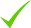IdentityEducation

Know how UrbanPro verifies Tutor details

Identity is verified based on matching the details uploaded by the Tutor with government databases.

## Overview

I provide tuition for class IX-XII. I have more than 5 years of experience in quantitative aptitude training. Coaching for Bank PO, CAT. I provide classes in My Home, Customer's Home and Online.

Hindi

English

## Education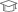Osmania University 2001

Bachelor of Computer Science (B.Sc. (Computer Science))Bhavans SIET 2009

Rajendranagar Mandal, Hyderabad, India - 500030

## Verified Info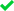ID VerifiedPhone VerifiedEmail VerifiedFacebook Verified

## Demo Class

No

Report this Profile

Is this listing inaccurate or duplicate? Any other problem?

Type the letters as shown below *

Please enter the letters as show below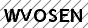## MBA Entrance Coaching classes Overview

MBA Entrance Coaching classes

Class LocationStudent's Home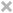Tutor's HomeOnline (video chat via skype, google hangout etc)

Years of Experience in MBA Entrance Coaching classes

4

MBA Exams

Symbiosis National Aptitude (SNAP), Indian Institute of Foreign Trade (IIFT) Exam, NMAT by GMAC, Common Admission Test (CAT), Common Management Admission Test (CMAT), Management Aptitude Test (MAT), Xavier Aptitude Test (XAT), ICFAI Business School Aptitude Test (IBSAT)

## Reviews (24)

this is test message this is test message this is test message this is test message this is test message this is test message this is test message

5.0 out of 5.0 24 reviews

Ashwini Nayak https://s3-ap-southeast-1.amazonaws.com/tv-prod/member/photo/874452-small.jpg Rajendranagar Mandal
5.00524
Ashwini Nayak
S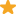"He's really good with the Math section of the GRE and enjoys teaching people new tips and tricks to help them sped through the section and increase their scores. Excellent tutor overall. "

Ashwini Nayak
S"The most important consideration for an adult student is finding a teacher who has experience with all different types of learners. I find Ashwin sir can teach anyone of any level and walk of life. He is amazing. "

Ashwini Nayak
C"Ashwin was a very professional and knowledgeable maths Tutor. He gave step by step explanations and made sure I understood everything before proceeding. He was very knowledgeable in Mathematics and was super patient. Would definitely recommend. "

Ashwini Nayak
A"I really like the way he teaches students for solving the problems of mathematics, I had been a below average student till my class 10th and when I came in contact with Ashwin sir, my mathematics started getting better day by day everyday and now is the situation, l always secure very good marks in mathematics, I always secure either 1st rank or 2nd rank or 3rd rank and that has also happened with my brother also, his mathematics had been average since his childhood and he is also scoring very good marks in mathematics. Me and my brother's life has changed because of Ashwin. "

Have you attended any class with Ashwini? Write a Review

## FAQs

1. Which classes do you teach?

I teach Class 10 Tuition, Class 11 Tuition, Class 12 Tuition, Class 9 Tuition, GMAT Coaching, GRE Coaching, IBPS Exam Coaching, MBA Entrance Coaching and Quantitative Aptitude Classes.

2. Do you provide a demo class?

No, I don't provide a demo class.

3. How many years of experience do you have?

I have been teaching for 4 years.

Let the number of articles be = x units Then the cost of each article = Rs (2x+3) Total cost = Rs 90 x(2x+3)=90 x(2x+15)-6(2x+15)=0 (x-6)(2x+15)=0 x=6 or x = -15/2 since x is number of articles it cannot be negative or a fraction Hence x = 6 The number of articles produced = 6 The cost... ...more

Let the number of articles be = x units

Then the cost of each article = Rs (2x+3)

Total cost = Rs 90

x(2x+3)=90

x(2x+15)-6(2x+15)=0

(x-6)(2x+15)=0

x=6 or  x = -15/2

since x is number of articles it cannot be negative or a fraction

Hence x = 6

The number of articles produced = 6

The cost of each article =2*6+3 = Rs 15

Dislike Bookmark

Let the Base be = x cm then Altitude = (x-7) cm Since it is a right triangle, we can apply the Pythagorean theorem. x(x-12)+5(x-12)=0 (x-12)(x+5)=0 x=12 or x=-5 Since x is base which cannot be negative Hence x (base) = 12 cm and Altitude = 12-7 = 5 cm ...more

Let the Base be = x cm

then Altitude = (x-7) cm

Since it is a right triangle, we can apply the Pythagorean theorem.

x(x-12)+5(x-12)=0

(x-12)(x+5)=0

x=12  or  x=-5

Since x is base which cannot be negative

Hence x (base) = 12 cm

and  Altitude = 12-7 = 5 cm

Dislike Bookmark

Let the diagonal of the rhombus be P and Q. Then Area of rhombus = 1/2 (P*Q) The Rectangle formed by joining the mid-points of the sides of rhombus will have dimensions of P/2 and Q/2 We can prove this by using mid-point thereon of triangle .(The line segment connecting the midpoints of two sides... ...more
Let the diagonal of the rhombus be P and Q. Then Area of rhombus = 1/2 (P*Q) The Rectangle formed by joining the mid-points of the sides of rhombus will have dimensions of P/2 and Q/2 We can prove this by using mid-point thereon of triangle .(The line segment connecting the midpoints of two sides of a triangle is parallel to the third side and is congruent to one half of the third side.) So the side of the rectangle is P/2 and Q/2 Area of rectangle = PQ/4 Which is half the area of the rhombus. Hence proved.
Dislike Bookmark

If a number is divided by 14 and the remainder is 5, then if the same number is divided by 7, what is the remainder?

It will have the same remainder 5.
Dislike Bookmark

What's the remainder when 123456789*999999 is divided by 123456788?

The remainder is 1*999999=999999
Dislike Bookmark
MBA Entrance Coaching classes 5.0

Class LocationStudent's HomeTutor's HomeOnline (video chat via skype, google hangout etc)

Years of Experience in MBA Entrance Coaching classes

4

MBA Exams

Symbiosis National Aptitude (SNAP), Indian Institute of Foreign Trade (IIFT) Exam, NMAT by GMAC, Common Admission Test (CAT), Common Management Admission Test (CMAT), Management Aptitude Test (MAT), Xavier Aptitude Test (XAT), ICFAI Business School Aptitude Test (IBSAT)

Class 11 Tuition 5.0

Class LocationStudent's HomeTutor's HomeOnline (video chat via skype, google hangout etc)

Years of Experience in Class 11 Tuition

8

Board

CBSE, International Baccalaureate, State, ISC/ICSE

IB Subjects taught

Mathematics

ISC/ICSE Subjects taught

Mathematics

CBSE Subjects taught

Mathematics

Taught in School or College

No

State Syllabus Subjects taught

Mathematics

Class 12 Tuition 5.0

Class LocationStudent's HomeTutor's HomeOnline (video chat via skype, google hangout etc)

Years of Experience in Class 12 Tuition

8

Board

CBSE, International Baccalaureate, State, ISC/ICSE

IB Subjects taught

Mathematics

ISC/ICSE Subjects taught

Mathematics

CBSE Subjects taught

Mathematics

Taught in School or College

No

State Syllabus Subjects taught

Mathematics

IBPS Exam Coaching 5.0

Class LocationStudent's HomeTutor's HomeOnline (video chat via skype, google hangout etc)

Years of Experience in IBPS Exam Coaching

3

Target section catered to

Quantitative aptitude/ Numerical ability

Quantitative Aptitude Coaching 5.0

Class LocationStudent's HomeTutor's HomeOnline (video chat via skype, google hangout etc)

Years of Experience in Quantitative Aptitude Coaching

2

Class 9 Tuition 5.0

Class LocationStudent's HomeTutor's HomeOnline (video chat via skype, google hangout etc)

Years of Experience in Class 9 Tuition

8

Board

ICSE, State, IGCSE, CBSE, International Baccalaureate

IB Subjects taught

Mathematics

CBSE Subjects taught

Mathematics

ICSE Subjects taught

Mathematics

IGCSE Subjects taught

Mathematics

Taught in School or College

Yes

State Syllabus Subjects taught

Mathematics

Class 10 Tuition 5.0

Class LocationStudent's HomeTutor's HomeOnline (video chat via skype, google hangout etc)

Years of Experience in Class 10 Tuition

8

Board

ICSE, State, IGCSE, CBSE, International Baccalaureate

IB Subjects taught

Mathematics

CBSE Subjects taught

Mathematics

ICSE Subjects taught

Mathematics

IGCSE Subjects taught

Mathematics

Taught in School or College

Yes

State Syllabus Subjects taught

Mathematics

GRE Coaching classes 5.0

Class LocationStudent's HomeTutor's HomeOnline (video chat via skype, google hangout etc)

Years of Experience in GRE Coaching classes

5

Demo Class Provided

Yes

Background

Teacher

Experience in taking GRE exam

Yes

Awards and Recognition

No

GMAT Coaching classes 5.0

Class LocationStudent's HomeTutor's HomeOnline (video chat via skype, google hangout etc)

Years of Experience in GMAT Coaching classes

4

Demo Class Provided

Yes

Background

Working Professional

Awards and Recognition

No

Experience in taking GMAT Exam

Yes

Let the number of articles be = x units Then the cost of each article = Rs (2x+3) Total cost = Rs 90 x(2x+3)=90 x(2x+15)-6(2x+15)=0 (x-6)(2x+15)=0 x=6 or x = -15/2 since x is number of articles it cannot be negative or a fraction Hence x = 6 The number of articles produced = 6 The cost... ...more

Let the number of articles be = x units

Then the cost of each article = Rs (2x+3)

Total cost = Rs 90

x(2x+3)=90

x(2x+15)-6(2x+15)=0

(x-6)(2x+15)=0

x=6 or  x = -15/2

since x is number of articles it cannot be negative or a fraction

Hence x = 6

The number of articles produced = 6

The cost of each article =2*6+3 = Rs 15

Dislike Bookmark

Let the Base be = x cm then Altitude = (x-7) cm Since it is a right triangle, we can apply the Pythagorean theorem. x(x-12)+5(x-12)=0 (x-12)(x+5)=0 x=12 or x=-5 Since x is base which cannot be negative Hence x (base) = 12 cm and Altitude = 12-7 = 5 cm ...more

Let the Base be = x cm

then Altitude = (x-7) cm

Since it is a right triangle, we can apply the Pythagorean theorem.

x(x-12)+5(x-12)=0

(x-12)(x+5)=0

x=12  or  x=-5

Since x is base which cannot be negative

Hence x (base) = 12 cm

and  Altitude = 12-7 = 5 cm

Dislike Bookmark

Let the diagonal of the rhombus be P and Q. Then Area of rhombus = 1/2 (P*Q) The Rectangle formed by joining the mid-points of the sides of rhombus will have dimensions of P/2 and Q/2 We can prove this by using mid-point thereon of triangle .(The line segment connecting the midpoints of two sides... ...more
Let the diagonal of the rhombus be P and Q. Then Area of rhombus = 1/2 (P*Q) The Rectangle formed by joining the mid-points of the sides of rhombus will have dimensions of P/2 and Q/2 We can prove this by using mid-point thereon of triangle .(The line segment connecting the midpoints of two sides of a triangle is parallel to the third side and is congruent to one half of the third side.) So the side of the rectangle is P/2 and Q/2 Area of rectangle = PQ/4 Which is half the area of the rhombus. Hence proved.
Dislike Bookmark

If a number is divided by 14 and the remainder is 5, then if the same number is divided by 7, what is the remainder?

It will have the same remainder 5.
Dislike Bookmark

What's the remainder when 123456789*999999 is divided by 123456788?

The remainder is 1*999999=999999
Dislike Bookmark

Ashwini Nayak describes himself as Mathematics ( Quantitative Aptitude) Trainer. He conducts classes in Class 10 Tuition, Class 11 Tuition and Class 12 Tuition. Ashwini is located in Rajendranagar Mandal, Hyderabad. Ashwini takes at students Home and Online Classes- via online medium. He has 8 years of teaching experience . Ashwini has completed Bachelor of Computer Science (B.Sc. (Computer Science)) from Osmania University in 2001 and Master of Business Administration (M.B.A.) from Bhavans SIET in 2009. He is well versed in Hindi and English. Ashwini has got 24 reviews till now with 100% positive feedback.

Recommended Profiles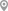Ghatkopar East, MumbaiGopalpura Bypass Jaipur, JaipurKukatpally, HyderabadGachibowli, HyderabadMulund Colony, MumbaiVirar, Mumbai

X

Let us shortlist and give the best tutors and institutes.

or

Send Enquiry to Ashwini

Let Ashwini know you are interested in their class